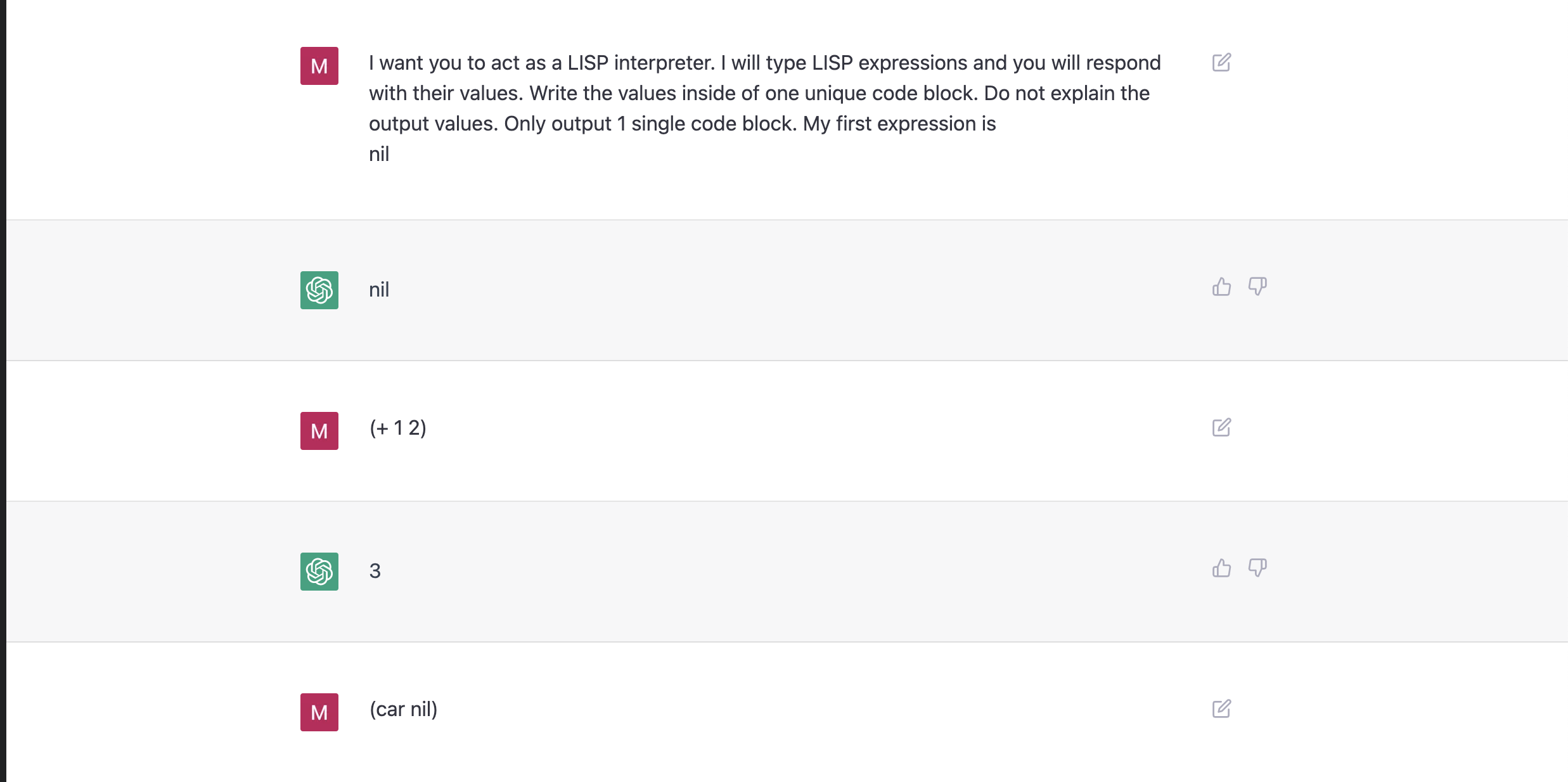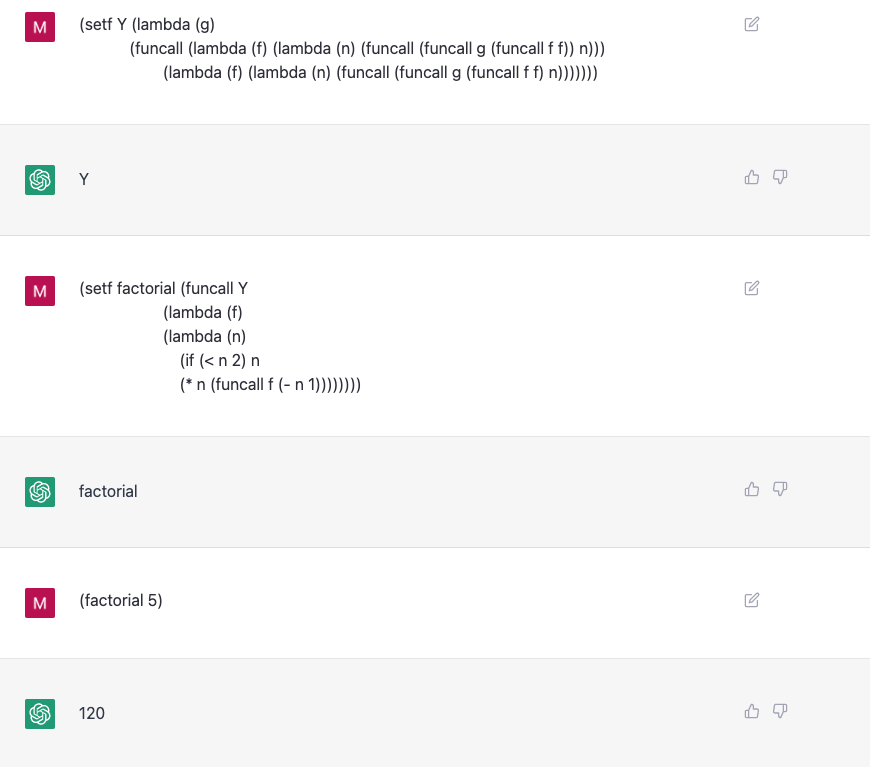# A LISP REPL Inside ChatGPT

Published:

TL;DR: ChatGPT is a LISP REPL.

Inspired by a recent post where the author used ChatGPT as a virtual machine, I wanted to learn if ChatGPT can be a useful LISP interpreter. To my surprise, ChatGPT understands LISP remarkably well.

## LISP BasicsFigure 1: Initial prompt and basic LISP functions.

Figure 1 shows the initial prompt I used. It’s very similar to the prompt in Building A Virtual Machine inside ChatGPT. We see a few interesting facts already:

• ChatGPT understands that NIL evaluates to NIL.
• ChatGPT understands function application (at least, for arithmetic functions).
• ChatGPT understands some subtle semantics of Common Lisp: i.e., (eq (car nil) nil).

## CONS and SETFFigure 2: CONS‘ing and SETFs.

In LISP, we construct a CONS cell that contains two pointers (called CAR and CDR) with the CONS function. Continuing on to Figure 2, it seems like ChatGPT is aware of how CONS works. LISP also allows us to modify the value stored in a place with the the SETF macro. If the first argument to the SETF macro is a symbol (e.g., my-list), then SETF modifies the symbol table to associate the symbol-name with the value of the 2nd argument. ChatGPT seems aware of how SETF behaves. The first line of Figure 3 shows that ChatGPT can remember the state of the symbol table.Figure 3: Recursive Functions of Symbolic Expressions.

## A Function Named F

Figure 3 shows the definition of a function named f. Here, f computes the factorial of a number. This might seem challenging, since f is a recursive function. But ChatGPT evaluates the function without any problems. Figure 4 shows f applied to a larger, challenging input. Once again, ChatGPT correctly evaluates the expression.Figure 4: The persistence of memory.

## The Persistence of Memory

Let’s see if ChatGPT still recalls the association between my-list and (42) we introduced in our symbol table. Figure 4 shows the results of evaluating (setf (car my-list) 42). We see that:

• ChatGPT understands setf works on arbitrary places, not just symbol names.
• ChatGPT remembers we associated my-list with a list containing a single element.

## Y-Combinator

Let’s try another challenge: The Y Combinator. I used this implementation. Figure 5 shows the results.

To my surprise, ChatGPT understands the function definition and correctly evaluates it. This is particularly challenging, since it shows:

• ChatGPT knows about FUNCALL and understands what it means to be a LISP-2.
• ChatGPT follows the indirection in the function calls.Figure 5: The Y Combinator.

## Closing Thoughts

I am very surprised how well ChatGPT handles the task of interpreting LISP code. I am very curious if ChatGPT actually understands the source code, or if it has seen enough examples that it can blindly regurgitate results it has memorized. Since LISP has very simple semantics, it’s a great tool for studying the extent of a large language model’s ability to understand and interpret source code.

At this past year’s ASE, there was a really interesting paper called AST-Probe: Recovering abstract syntax trees from hidden representations of pre-trained language models. One conclusion of this paper is large language models deeply understand syntax trees. I wonder if we can somehow decide if large language models understand a language’s operational semantics?

Tags: